Private GMAT Tutoring in NYC & Online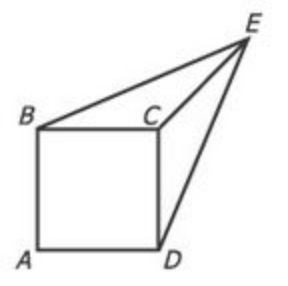In the figure above, each side of square ABCD has length 1, the length of line segment CE is 1, and the length of line segment BE is equal to the length of line segment DE. What is the area of the triangular region BCE?

(A) 1/3
(B) √2/4
(C) 1/2
(D) √2/2
(E) 3/4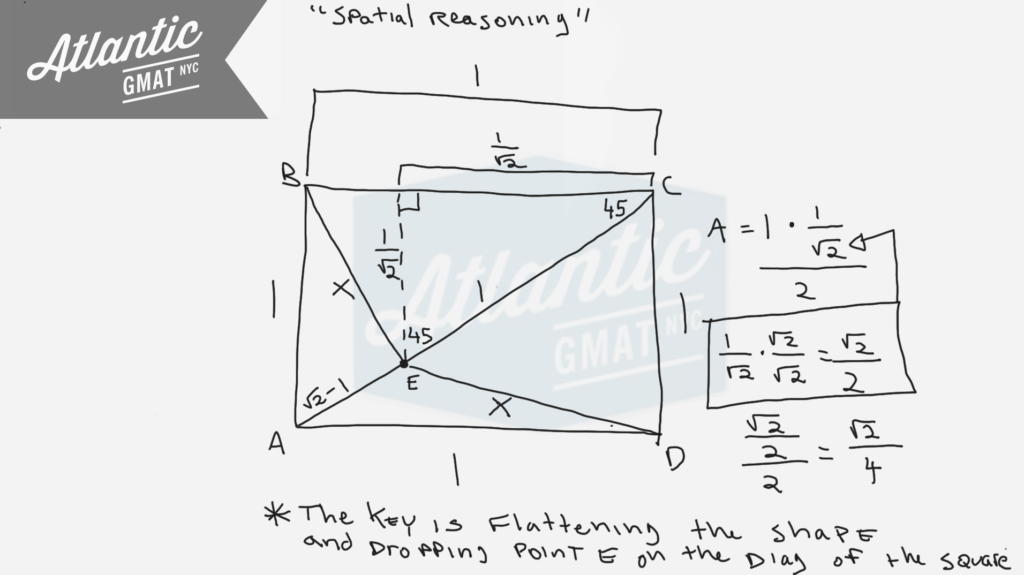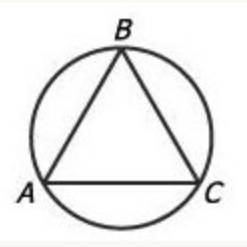In the figure above, equilateral triangle ABC is inscribed in the circle. If the length of arc ABC is 24, what is the approximate diameter of the circle?

(A) 5
(B) 8
(C) 11
(D) 15
(E) 19

It's key to know that an equilateral triangle has equal sides and equal angles measuring 60 degrees each. So each angle of an equilateral triangle inscribed in a circle cuts off 1/3 of the circle. Why is that? Review the basics! An angle on the circumference of a circle cuts off twice that angle's measure. So a 60 degree angle on the edge of a circle cuts off 120 degrees or 1/3 of a circle (120/360 degrees). A 60 degree angle in the center of a circle only cuts off 60 degrees (it doesn't have the extra space to open up). Examples below to illustrate this. Also, there's a special case for triangles that have the diameter of a circle as their base. Because the diameter is a 180 degree central angle, the angle opposite on the circumference of the circle must be 90 degrees. Not relevant for this question but good to know.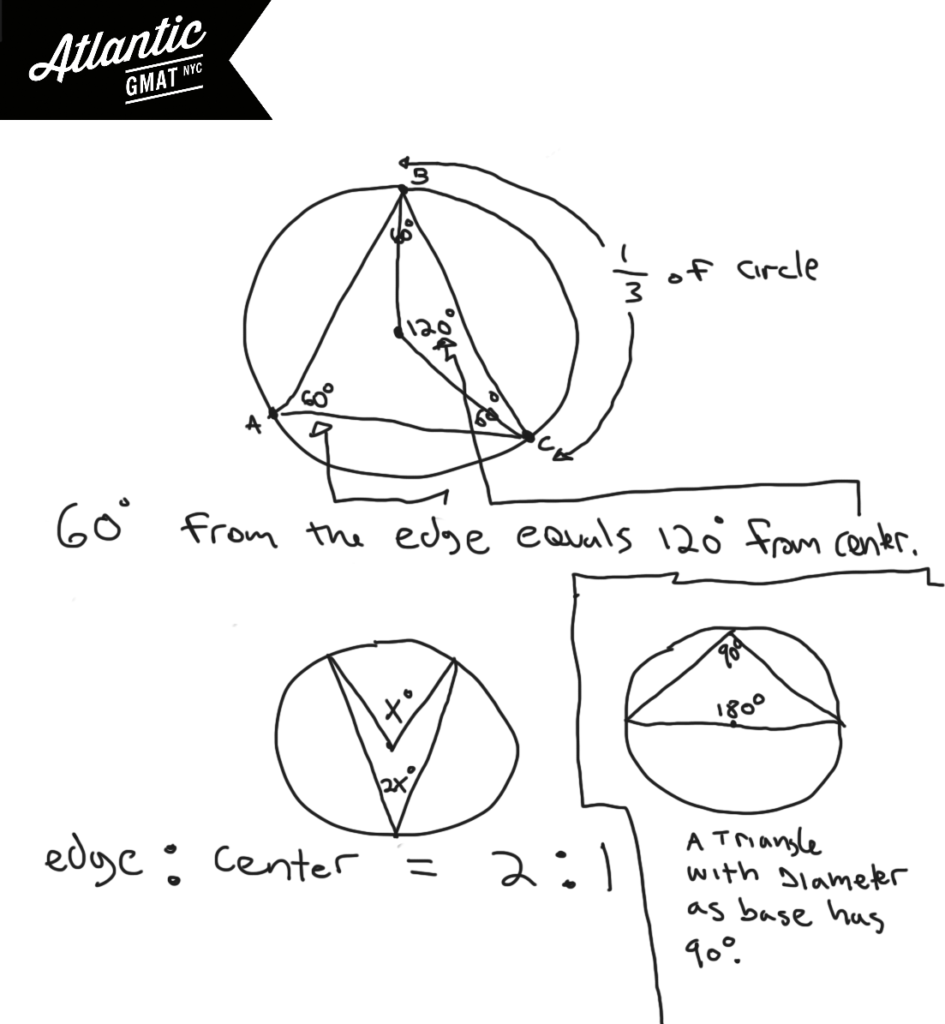How much of the circle is arc ABC? It's covering the same part of the circumference as two of the 60 degree angles from triangle ABA. Each of those angles represent 1/3 of the circle so arc ABC is 2/3 of the circle. If 2/3 is 24, 1/3 of the circle's circumference is 12, and the entire circumference is 36. So Pi*diameter = circumference. Pi*D = 36. D = 36/Pi. D = 36/3.14. A little less than 12. 11 is by far the closest answer choice. A little rusty feeling on these GMAT geometry rules? The rules are the easy part of GMAT studying. Get your fundamentals in shape ASAP. Avoid churning through questions before getting your basics GMAT quant organized. You don't want to waste good material and it's much harder to focus on the basics while working on tougher/trickier GMAT questions. Comment with any questions or additions. Happy studies!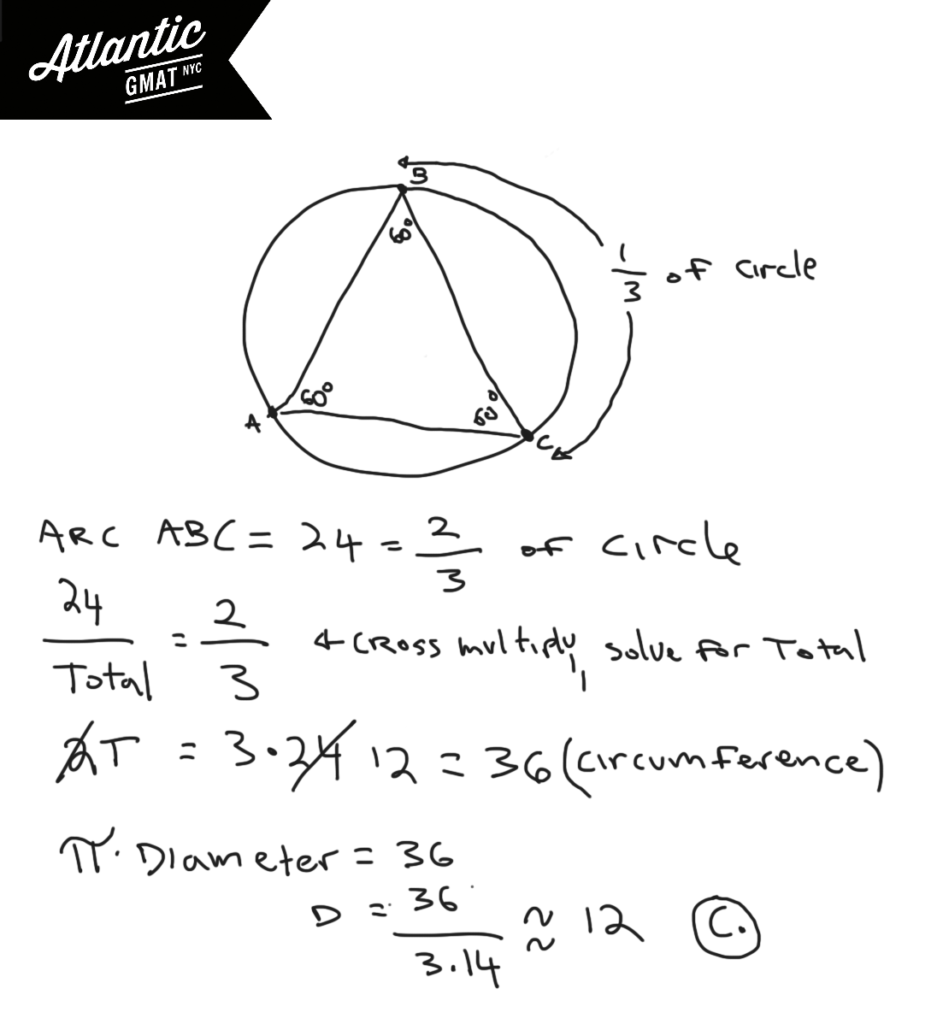A right circular cone is inscribed in a hemisphere so that the base of the cone coincides with the base of the hemisphere. What is the ratio of the height of the cone to the radius of the hemisphere?

(A) √3:1
(B) 1:1
(C) 1/2/1
(D) √2:1
(E) 2:1

# GMAT Question of the Day - Data Sufficiency - Geometry/Triangles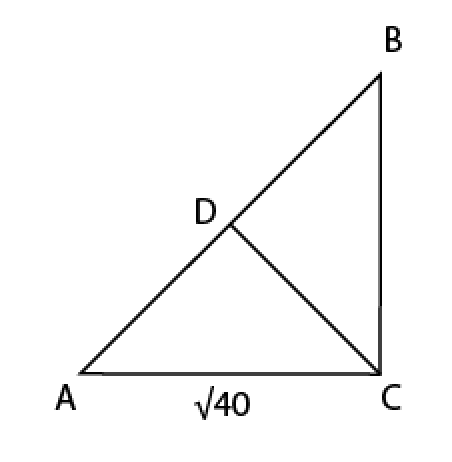If the length of side AC is equal to the length of side BC and the length of segment AD is equal to the length of segment BD is the length of segment CD less than half the value of the length of side AB?

1) The measure of angle ACB is not less than 90

2) The area of triangle ABC is 20

## GMAT Question of the Day Solution

This question of the day is tricky but not tough if you stay organized. From the diagram and the given information we know that this is an isosceles triangle. Is it a right isosceles triangle? We don't know. However, if it is a right isosceles triangle then we can certainly calculate the values for all of the sides and determine the relationship between AB and CD. So one of the ways of answering this question comes down to determining whether triangle ABC is in fact a 45-45-90 triangle.

Statement 1) Not less than 90 means greater than or equal to 90. That means that we could have a right isosceles triangle. If we do have a right isosceles we can calculate the values for all of the sides based on the ratio 1:1:√2. That would make side CD exactly half of the value of AB. Meaning that we could answer NO to the question. Can we answer YES? Well, if the angle ABC is greater than 90 the hypotenuse will get longer while CD gets shorter. Hence CD will be less than half of AB (you can see this clearly in the diagram). So the answer would be YES. Both YES and NO means Insufficient.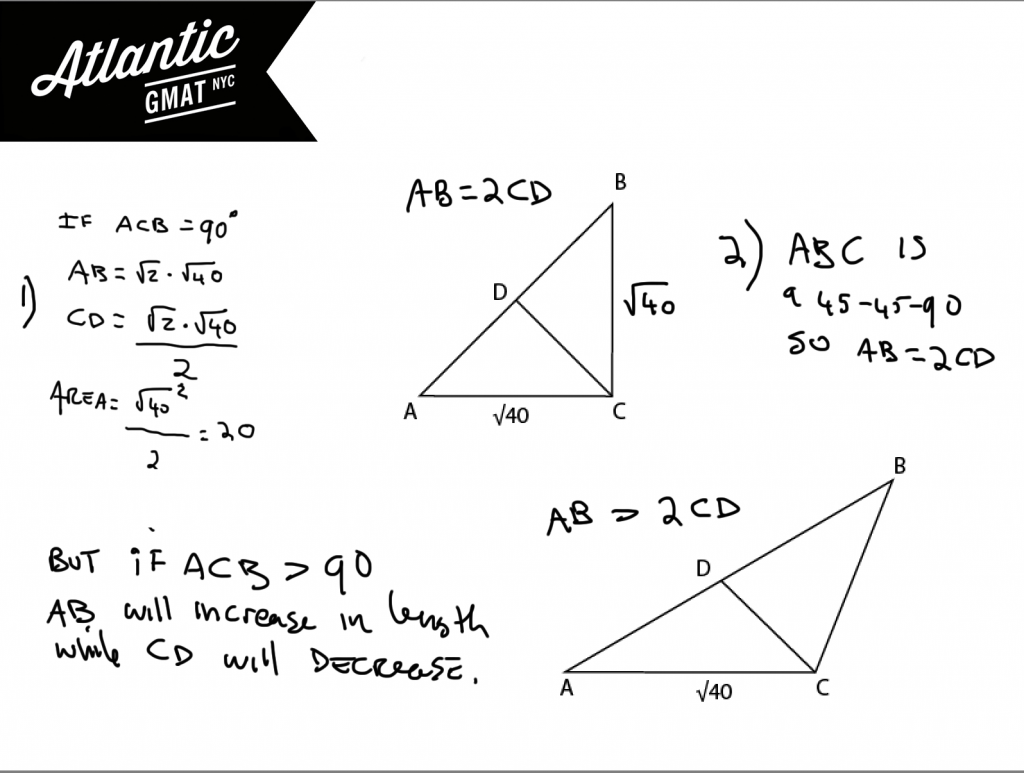2) It would have been great to have calculated the 45-45-90 scenario in advance. We shouldn't have assumed that this was a 45-45-90 but because it is an easy scenario to calculate it is worth doing. This is something that I always try to encourage in my GMAT tutoring students: to take simple steps to create more useful information.

This area proves that the triangle is in fact a right isosceles so we can determine the values for all of the sides. Sufficient.

# CONTACT

Atlantic GMAT

405 East 51st St.

NY, NY 10022

(347) 669-3545

info@AtlanticGMAT.com